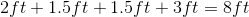# ISEE Middle Level Math : How to find the perimeter of a trapezoid

## Example Questions

### Example Question #1 : How To Find The Perimeter Of A Trapezoid

The perimeter of the following trapezoid is equal to 23 cm. Solve for. (Figure not drawn to scale.)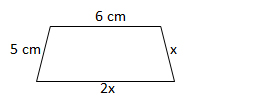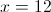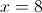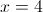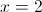Explanation:

The perimeter is equal to the sum of all of the sides.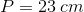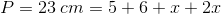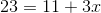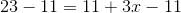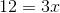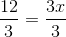### Example Question #42 : Geometry

Find the perimeter of the trapezoid: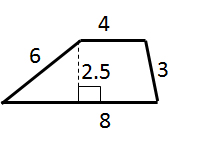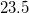Explanation:

The perimeter of any shape is equal to the sum of the lengths of its sides: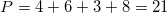### Example Question #2 : How To Find The Perimeter Of A Trapezoid

You recently bought a new bookshelf with a base in  the shape of an isosceles trapezoid. If the small base is 2 feet, the large base is 3 feet, and the arms are 1.5 feet, what is the perimeter of the base of your new bookshelf?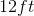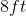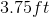Cannot be determined from the information provided.Explanation:

You recently bought a new bookshelf with a base in  the shape of an isosceles trapezoid. If the small base is 2 feet, the large base is 3 feet, and the arms are 1.5 feet, what is the perimeter of the base of your new bookshelf?

To find the perimeter of a bookshelf, we need to add up the lengths of the sides.

We know the two bases, we just need to add the lengths of the arms.